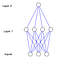# How to build a multi-layered neural network in Python

In , thanks to an , I learned how to build a neural network for the first time. It was super simple. 9 lines of Python code modelling the behaviour of a single neuron.

But what if we are faced with a more difficult problem? Can you guess what the ‘?’ should be?

The trick is to notice that the third column is irrelevant, but the first two columns exhibit the behaviour of a . If either the first column or the second column is 1, then the output is 1. However, if both columns are 0 or both columns are 1, then the output is 0.

So the correct answer is 0.

However, this would be too much for our single neuron to handle. This is considered a “nonlinear pattern” because there is no direct one-to-one relationship between the inputs and the output.

Instead, we must create an additional hidden layer, consisting of four neurons (Layer 1). This layer enables the neural network to think about combinations of inputs.A diagram of our neural network. The blue lines represent synaptic connections between neurons. The diagram was automatically generated using: https://github.com/miloharper/visualise-neural-network

You can see from the diagram that the output of Layer 1 feeds into Layer 2. It is now possible for the neural network to discover correlations between the output of Layer 1 and the output in the training set. As the neural network learns, it will amplify those correlations by adjusting the weights in both layers.

In fact, image recognition is very similar. There is no direct relationship between pixels and apples. But there is a direct relationship between combinations of pixels and apples.

The process of adding more layers to a neural network, so it can think about combinations, is called “deep learning”. Ok, are we ready for the Python code? First I’ll give you the code and then I’ll explain further.

Also available here:

This code is an adaptation from my previous neural network. So for a more comprehensive explanation, .

What’s different this time, is that there are multiple layers. When the neural network calculates the error in layer 2, it propagates the error backwards to layer 1, adjusting the weights as it goes. This is called “back propagation”.

Ok, let’s try running it using the Terminal command:

python main.py

You should get a result that looks like this:

First the neural network assigned herself random weights to her synaptic connections, then she trained herself using the training set. Then she considered a new situation [1, 1, 0] that she hadn’t seen before and predicted 0.0078876. The correct answer is 0. So she was pretty close!

You might have noticed that as my neural network has become smarter I’ve inadvertently personified her by using “she” instead of “it”.

That’s pretty cool. But the computer is doing lots of matrix multiplication behind the scenes, which is hard to visualise.

Written by

Written by

## Milo Spencer-Harper

#### Studied Economics at Oxford University. Founder of www.magimetrics.com, acquired by www.socialstudies.io Interested in machine learning.